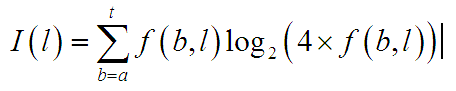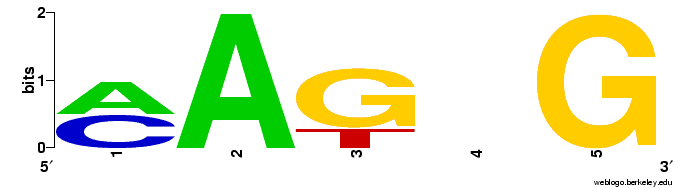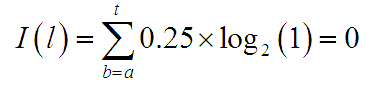# p values and baysian scores

## Binding probability: The simplest case.

Consider a transcription factor that only binds to the sequence ATATG.

Using this information it is possible to calculate the p-value for any given sequence which is an indication of whether the transcription factor will bind. In this simple case, if the sequence is ATATG then the p value is 1, for any other sequence it is 0.

## Binding probabilities: Position Weight Matrixes (PSSM or PWM)

Consider a simple binding region where the binding sequences that have been found are AATAG, AAGTG, CAGCG CAGGG. The simplest assumption is that these sequences are reprentative of the range nucleotide sequences that the transcription factor binds. The frequency distribution of nucleotides by position is:

 Position A C G T Information Content 1 2 2 0 0 1 2 4 0 0 0 2 3 0 0 3 1 1.19 4 1 1 1 1 0 5 0 0 4 0 2

In this case, it is less obvious how to calculate the probability that a transcription factor will bind to any arbitrary sequence.

One starting point is to consider the information content at each location. If we assume that there would otherwise be an equal probability of each of the four nucleotides at each position then I(l), the information content at position l can be represented as:where I(l) is the information content at position l and f(b,l) is the frequency of nucleotide b at position l.

This equation is presented in a number of forms in different contexts (Schneider and Stephens 1990; Kel, Gossling et al. 2003) but they can all be converted into this form. The information content is used to define the heights of the letters in each nucleotide position when representing the position weight matrix in logo form:Note that there is an implicit assumption that all nucleotides are equally likely, resulting in the frequencies being multiplied by 4 before taking logs, such that in the case of the nucleotides being equally probable the equation is, that is to say that there is no information content. If the background nucleotide distribution was different (e.g. this was an AT rich region) then this assumption is not valid, in that a position where a,t,c or g were equally likely would be unusual given the background sequence.

## Calculating the biobase binding score

The weight matrix can then be used to calculate the a biobase binding score for any arbitrary DNA sequence. The standard basis for this is to calculate the sum across the sequence of the product of the frequency of occurrence of the nucleotide and the information content. In the above example, if the DNA sequence was AATTC, the score would be calculated as:

 A C G T Information Sequence to be 'scored' Biobase nucleotide 'score' 2 2 0 0 1 A 2/4*1 = 0.5 4 0 0 0 2 A 4/4*2 = 2 0 0 3 1 1.19 T 1/4*1.19=0.29 1 1 1 1 0 T 1/4*0=0 0 0 4 0 2 C 0/4*2=0 Total = 2.79

There remains the issue of determining the significance of the score. One approach used in the Match algorithm (Kel, Gossling et al. 2003) and the BIFA tool is to normalise the score to fit within the range 0 to 1 where 0 is the score of the worst possible match to the PSSM (e.g. GGAAA, scoring 0 in the above example) and 1 is the best possible match (AAGAG, scoring 5.39 in the above example), such that 2.79 is normalised to 2.79/5.39 = 0.51.

## Assessing the binding probability

The next step is to assess the significance of this score, which normally amounts to assessing the significance of finding a specific sequence given the PWM and the background DNA statistics.

The technique used in the bifa tool is to calculate the score and log likelyhood for every single possible nucleotide sequence using dynamic programming methods, ie calcalate the scores/likelyhoods for the four possible values of the first nucleotide, and then for each of these scores calculate what the new scores/likelyhoods will be for each possible value of the second nucleotide, and so on for each nucleotide. The number of scores increases exponentially, so similar scores are combined as necessary. This can then be used to create a map that shows how scores (which range between 0 and 1) map to likelyhoods, in 0.01 score increments.

Kel, A. E., E. Gossling, et al. (2003). "MATCH: A tool for searching transcription factor binding sites in DNA sequences." Nucleic Acids Res 31(13): 3576-9.

Schneider, T. D. and R. M. Stephens (1990). "Sequence logos: a new way to display consensus sequences." Nucleic Acids Res 18(20): 6097-100.